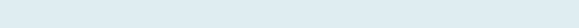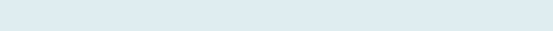# Count Formula for Specific Text

After reading this article you will be able to count formula for Text only, even though cell range contain text and numbers (both) in a Data set. The return numbers are only a count of Texts it ignores any number, date or any numerical data sets.

Query

We have two columns, Student Name and Marks. Our task is to know how many students Absent in column C.

Formula to implement:

=COUNTA(C3:C11)-COUNT(C3:C11)

Functions applicable:

We will be creating a COUNTA and COUNT formula to count text in Excel:

• COUNTA: Returns the count of numbers and text within the reference list of arguments ignoring blank cells.
• COUNT: Function compute the count of numbers within the reference list of arguments ignoring the text and blank cells.

Formula Explanation (step by step)

You can also evaluate the formula, just click F9 function key in Excel.

Let’s break the formula into multiple small steps to understand its application.

First formula:

=COUNTA(C3:C11)

Syntax: COUNTA(value1, [value2], …)

Step 1:Result: 9

It counts all the values including text in the given range (C3 to C11)

Second Formula:

=COUNT(C3:C11)

Syntax: COUNT(value1, [value2], …)

Step 2:Result: 6

Function returns count of cells that contain numbers (6) in the given range (C3 to C11)

Step 3: COUNTA(C3:C11)-COUNT(C3:C11)

Result: 3

(9-6) = 3

As text “Absent” comes three times in the given data range. so, it returns 3.